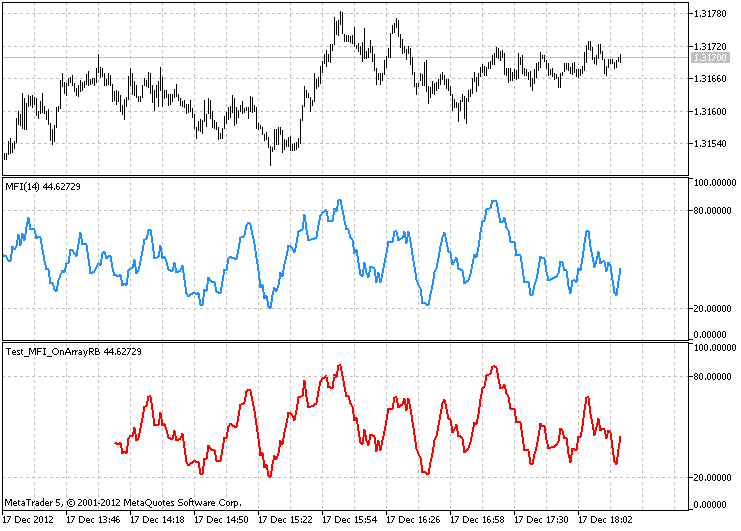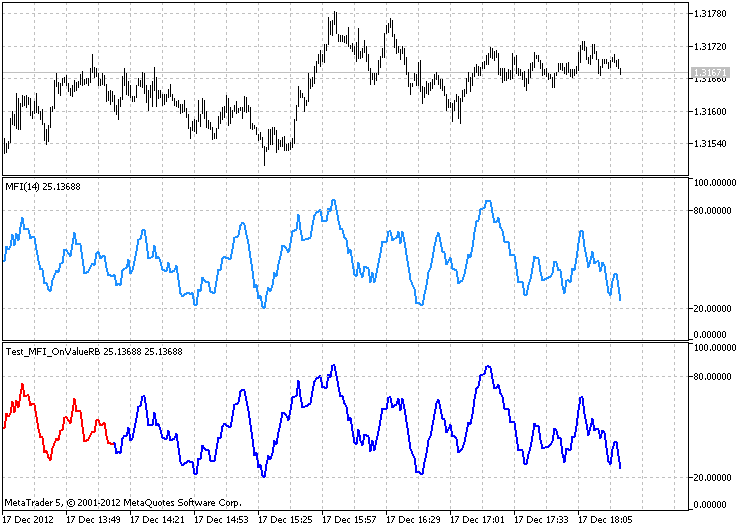Interesting script?
So post a link to it -
let others appraise it

You liked the script? Try it in the MetaTrader 5 terminal# The class for drawing the MFI using the ring buffer - indicator for MetaTrader 5

Views:
4303
Rating:
Published:
2013.01.08 14:46
Updated:
2016.11.22 07:32

Description

The CMFIOnRingBuffer class is designed to calculate the technical indicator Money Flow Index (Money Flow Index, MFI) using the algorithm of  the ring buffer

Declaration

`class CMFIOnRingBuffer : public CArrayRing`

Title

`#include <IncOnRingBuffer\CMFIOnRingBuffer.mqh>`

File of the CMFIOnRingBuffer.mqh class must be placed in IncOnRingBuffer folder that need to be established in MQL5\Include\. Two files with the examples used by the class from this folder are attached to the description. File with the class of the ring buffer and the class of Moving Average also must be in this folder.

Class methods

```//--- initialization method:
bool Init(                                             // if error it returns false,
// if successful - true
int                 period         = 14,            // the period of the MFI
ENUM_MA_METHOD      method         = MODE_SMA,      // the method of smoothibg
ENUM_APPLIED_PRICE  applied_price  = PRICE_TYPICAL, // price used for calculation
ENUM_APPLIED_VOLUME applied_volume = VOLUME_TICK,   // volume used for calculation
int                 size_buffer    = 256,           // the size of the ring buffer
bool                as_series      = false          // true, if a time series, otherwise - false
);```
```//--- the method of calculation based on the time series or indicator buffers:
int MainOnArray(                  // returns the number of the processed elements
const int     rates_total,     // the size of the arrays
const int     prev_calculated, // processed elements on the previous call
const double& open[],          // open prices
const double& high[],          // the maximum prices
const double& low[],           // the minimum prices
const double& close[],         // close prices
const long&   tick_volume[],   // tick volume
const long&   volume[]);       // stock volume
);```
```//--- method of calculation on the basis of the separate series elements of the array
double MainOnValue(              // returns the MFI value for the set element (bar)
const int    rates_total,     // the size of the array
const int    prev_calculated, // processed elements of the array
const int    begin,           // from where the significant data of the array starts
const double open,            // price of bar opening
const double high,            // the maximum price of the bar
const double low,             // the minimum price of the bar
const double close,           // price of bar closing
const long   tick_volume,     // tick volume of the bar
const long   volume,          // stock volume of the bar
const int    index            // the element (bar) index
);```
```//--- the methods of access to the data:
int                 BarsRequired(); // Returns the necessary number of bars to draw the indicator
string              Name();         // Returns the name of the indicator
int                 Period();       // Returns the period
string              Method();       // Returns the method in the form of the text line
ENUM_APPLIED_PRICE  Price();        // Returns the type of the used price
ENUM_APPLIED_VOLUME Volume();       // Returns the type of the used volume
int                 Size();         // Returns the size of the ring buffer```

To get the calculated data of the indicator from the ring buffer is possible as from the usual array. For example:

```//--- the class with the methods of calculation of the MFI indicator:
#include <IncOnRingBuffer\CMFIOnRingBuffer.mqh>
CMFIOnRingBuffer mfi;

...

//+------------------------------------------------------------------+
//| Custom indicator iteration function                              |
//+------------------------------------------------------------------+
int OnCalculate(const int rates_total,
const int prev_calculated,
const datetime& time[],
const double& open[],
const double& high[],
const double& low[],
const double& close[],
const long& tick_volume[],
const long& volume[],
{
//--- calculation of the indicator based on time series:
mfi.MainOnArray(rates_total,prev_calculated,open,high,low,close,tick_volume,volume);

...

//--- use data from the ring buffers "mfi",
//    for example, copy data in the indicator buffer:
for(int i=start;i<rates_total && !IsStopped();i++)
MFI_Buffer[i] = mfi[rates_total-1-i]; // indicator line

...

//--- return value of prev_calculated for next call:
return(rates_total);
}```

Please note that indexing in the ring buffer is the same as in the time series.

Examples

1. The Test_MFI_OnArrayRB.mq5 file calculates the indicator based on the price time series. The MainOnArray() method application is demonstrated
2. The Test_MFI_OnValueRB.mq5 file demonstrates the use of the MainOnValue() method. At first the MFI indicator is calculated and drawn. Then on the basis of this ring buffer of this indicator one more MFI indicator is drawn.The result of the work of the Test_MFI_OnArrayRB.mq5 with the size of the ring buffer of 256 elementsThe result of the work of the Test_MFI_OnValueRB.mq5 with the size of the ring buffer of 256 elements

When writing code the developments of MetaQuotes Software Corp.Integer and GODZILLA were used.

Translated from Russian by MetaQuotes Software Corp.
Original code: https://www.mql5.com/ru/code/1395The class for drawing the AMA using the ring buffer

The class is designed for calculation of the technical indicator Adaptive Moving Average (Adaptive Moving Average, AMA) using the algorithm of the ring buffer.RD-TrendTrigger

The oscillator using T3 averaging from the Technical Analysis of Stocks and Commodities (Dec. 2004).MACDWaterlineCrossExpectator, the effectiveness of a MACD system

This is the classical trading system which consists in buying when MACD crosses above the waterline line and selling when crosses below it. This EA works along with a monetary management system which has a positive mathematical expectation.T3MACO

The oscillator using T3 averaging.